Refer to our Texas Go Math Grade 5 Answer Key Pdf to score good marks in the exams. Test yourself by practicing the problems from Texas Go Math Grade 5 Module 12 Assessment Answer Key.

Vocabulary

Choose the best term from the box.

Question 1.
A ___ has a length, width, and height of 1 unit. (p. 435)
We know that,
A “Rectangular Prism” has a length, width, and height of 1 unit
Hence, from the above,
We can conclude that
The best term that is suitable for the given statement is: Rectangular Prism

Question 2.
Volume is measured in ____. (p. 441)
We know that,
The volume is measured in “Cubic Units”
Hence, from the above,
We can conclude that
The best term that is suitable for the given statement is: Cubic Units

Concepts and Skills

Count the number of cubes used to build each solid figure. TEKS 5.6.A

Question 3.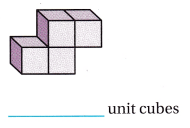The given figure is: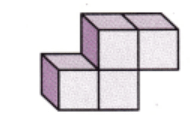Now,
From the given figure,
We can observe that
There are 4 cubes in the given figure
Hence, from the above,
We can conclude that
The number of cubes present in the given figure is: 4 cubes

Question 4.The given figure is: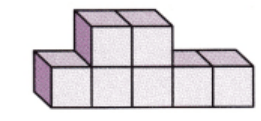Now,
From the given figure,
We can observe that
There are 7 cubes in the given figure
Hence, from the above,
We can conclude that
The number of cubes present in the given figure is: 7 cubes

Question 5.The given figure is:Now,
From the given figure,
We can observe that
There are 4 cubes in the given figure
Hence, from the above,
We can conclude that
The number of cubes present in the given figure is: 4 cubes

Find the volume. TEKS 5.6, A. 5.6.B

Question 6.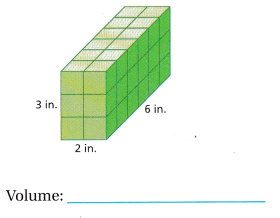The given figure is: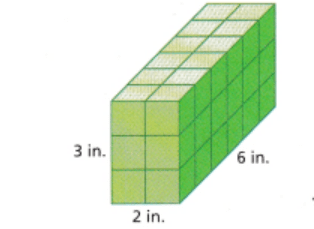Now,
We know that,
Volume = Length × Width × Height
So,
The volume of the given figure = 2 × 3 × 6
= 36 in.³
Hence, from the above,
We can conclude that
The volume of the given figure is: 36 in³

Question 7.The given figure is: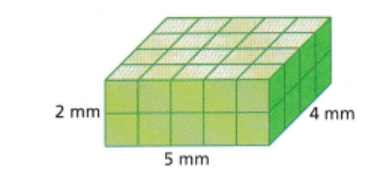Now,
We know that,
Volume = Length × Width × Height
So,
The volume of the given figure = 2 × 5 × 4
= 40 mm³
Hence, from the above,
We can conclude that
The volume of the given figure is: 40 mm³

Question 8.The given figure is:Now,
We know that,
Volume = Length × Width × Height
So,
The volume of the given figure = 3 × 5 × 7
= 105 ft³
Hence, from the above,
We can conclude that
The volume of the given figure is: 105 ft³

Question 9.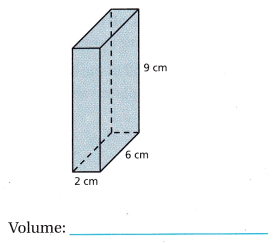The given figure is: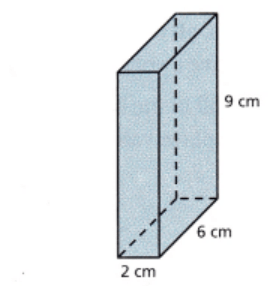Now,
We know that,
Volume = Length × Width × Height
So,
The volume of the given figure = 2 × 6 × 9
= 108 cm³
Hence, from the above,
We can conclude that
The volume of the given figure is: 108 cm³

Texas Test Prep

Question 10.
Sharri packed away her old summer clothes in a storage tote that had a length of 3 feet, a width of 4 feet, and a height of 3 feet. What was the volume of the tote that Sharri used? TEKS 5.6.B
(A) 36 cu ft
(B) 21 cu ft
(C) 24 cu ft
(D) 10 cu ft
It is given that
Sharri packed away her old summer clothes in a storage tote that had a length of 3 feet, a width of 4 feet, and a height of 3 feet
Now,
According to the given information,
The volume of the tote that Sharri used = 3 × 4 × 3
= 36 ft³
Hence, from the above,
We can conclude that
The volume of the tote that Sharri used is:Question 11.
A manufacturing company constructs a shipping box to hold its cereal boxes. Each cereal box has a volume of 40 cubic inches. If the shipping box holds 8 layers with 4 cereal boxes in each layer, what is the volume of the shipping box? TEKS 5.6.B
(A) 160 cu in.
(B) 320 cu in.
(C) 480 cu in.
(D) 1,280 cu in.
It is given that
A manufacturing company constructs a shipping box to hold its cereal boxes. Each cereal box has a volume of 40 cubic inches and the shipping box holds 8 layers with 4 cereal boxes in each layer
So,
According to the given information,
The total number of cereal boxes = 4 × 8 = 32 cereal boxes
Now,
The volume of the shipping box = 40 × 32
= 1,280 in³
Hence, from the above,
We can conclude that
The volume of the shipping box is: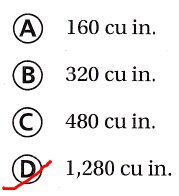Question 12.
Angela is deciding which of two aquariums to buy. One aquarium has a base that is 18 inches by 14 inches and a height that is 12 inches. The other aquarium has a base that is 25 inches by 9 inches and a height that is 14 inches. What is the difference between the volumes of the two aquariums? TEKS 5.6.B
(A) 3,024 cu in.
(B) 3,150 cu in.
(C) 126 cu in.
(C) 150 cu in.
It is given that
Angela is deciding which of two aquariums to buy. One aquarium has a base that is 18 inches by 14 inches and a height that is 12 inches. The other aquarium has a base that is 25 inches by 9 inches and a height that is 14 inches
Now,
According to the given information,
The volume of the first aquarium = 18 × 14 × 12
= 3,024 inches³
The volume of the second aquarium = 25 × 9 × 14
= 3,150 inches³
Now,
The difference between the volumes of the two aquariums = 3,150 – 3,024
= 126 inches³
Hence, from the above,
We can conclude that
The difference between the volumes of the two aquariums is:Question 13.
Roberto wants to build a wooden box with a volume of 8 cubic feet. How many different boxes, all with whole-number dimensions and a different-size base, will have a volume of 8 cubic feet? TEKS 5.6.B
Be sure to use the correct place value.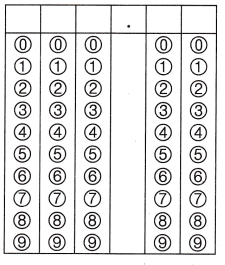It is given that
Roberto wants to build a wooden box with a volume of 8 cubic feet
Now,
We know that,
The factors of 8 are:
1, 2, 4, and 8
So,
The different dimensions for the volume of a wooden box built by Roberto is:
(1 × 8) × 1 = 8
(2 × 4) × 1 = 8
(1 × 4) × 2 = 8
(4 × 1) × 2 = 8
(1 × 1) × 8 = 8
(8 × 1) × 1 = 8
(4 × 2) × 1 = 8
Hence, from the above,
We can conclude that
The number of different boxes that have a volume of 8 cubic feet is:Scroll to Top